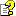SiteView help
Equation For Strain Bridge

What is a Strain Gauge
A strain gauge (also strain gage) is a device used to measure the strain of an object.
The gauge is attached to the object by a suitable adhesive. As the object is deformed, the foil is deformed, causing its electrical resistance to change. This resistance change, usually measured using a Wheatstone bridge, is related to the strain by the quantity known as the gauge factor.
The gauge factor GF is defined as: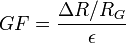Where:
?R is the change in resistance caused by strain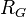is the resistance of the undeformed gauge, and e is strain.

A Wheatstone bridge is an electrical circuit used to measure an unknown electrical resistance by balancing two legs of a bridge circuit. The Wheatstone bridge consists of four resistances (R1, R2, R3 and R4), an excitation voltage and an output voltage. Generally, one or more of the resistances are variable and change in accordance with some physical phenomenon, such as strain in this case. The Wheatstone bridge then converts this change in resistance to a change in voltage.

There are 3 configurations are used - quarter bridge, half bridge or full bridge.

Quarter Bridge Circuit:
Only one active strain gauge (Rg) is used as shown below. R3 is the inactive gauge, which is identical to the active gauge but does not encounter any mechanical strains and is used for compensating the temperature effect. The other two arms contain fixed resistors.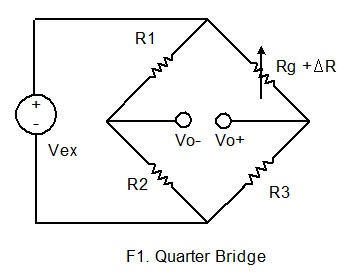Half Bridge Circuit:
If the dummy gauge in the above figure is replaced by an active gauge, as shown below, the resulting arrangement is called a half bridge. The half bridge has advantages for temperature compensation and higher bridge sensitivity over the quarter bridge so that small strain levels can be detected more accurately.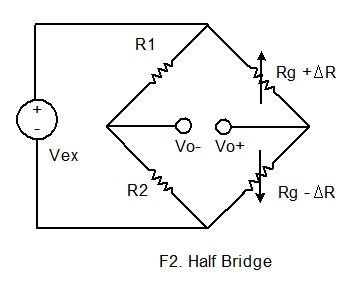Full Bridge Circuit:
If four active gauges are employed in the circuit, the arrangement is called Full Bridge as shown below.  It is automatically temperature compensated when all four active gauges bonded on the same material, and the highest sensitivity is obtained.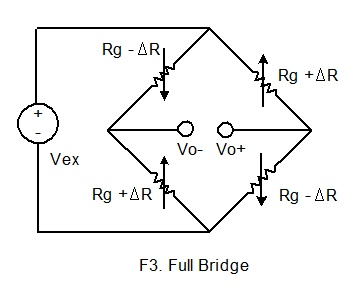Connect Strain Gauge to a Data Logger
iLog Srtrain Gauge/Bridge data logger supports six-wire configuration when connecting to a wheatstone bridge circuit. The following wirings are for SiteView built-in Strain Gauge equations:

Wiring for Quarter Bridge Circuit: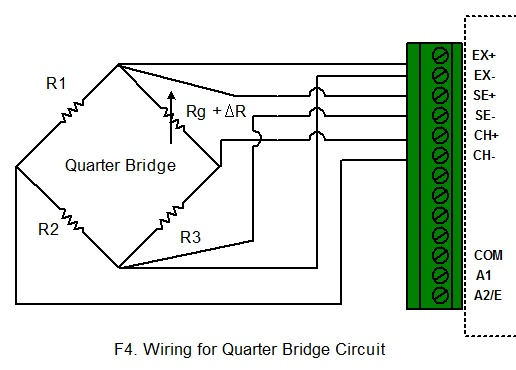This circuit is for equation QuarterBridge. When configure the logger, choose QuarterBridge equation and click Change GF to change the Gauge Factor.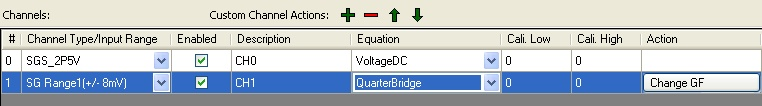Wiring for Half Bridge Circuit: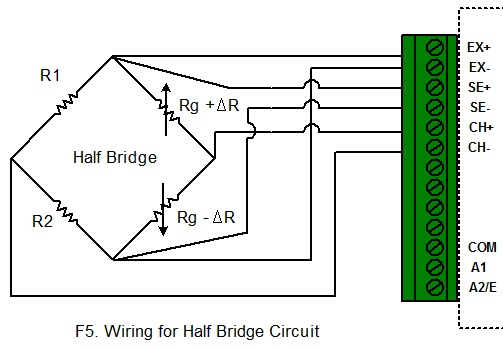This circuit is for equation HalfBridge. When configure the logger, choose HalfBridge equation and click Change GF to change the Gauge Factor.

Wiring for Full Bridge Circuit: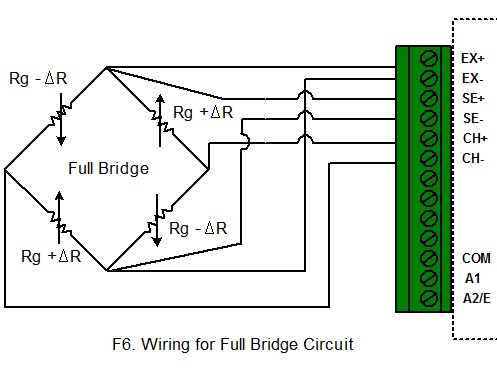This circuit is for equation FullBridge. When configure the logger, choose FullBridge equation and click Change GF to change the Gauge Factor.

Other Bridge Circuit:
If your bridge circuit is not included in the above configurations, you may need to write your own equation. SiteView includes a custom equation BridgeSample for your reference. The source code  looks like:

// Sample Equation for iLog Strain Gauge/Bridge

public double SampleBridge(double Input)

{

//Change it according to your curcuit

double GF = 1;//Gauge Factor

//The voltage of the bridge output

double Vo = Input;

//The voltage of the Excitation sense channel

double Vex = Channels.Measurement;

/* this block is for half bridge circuit

double strain = -2 * Vo / (Vex * GF);

return strain;

*/

/* this block is for full bridge circuit

double strain = -Vo / (Vex * GF);

return strain;

*/

/* this block is for quarter bridge circuit */

double strain = -4 * Vo / ((2 * Vo + Vex) * GF);

return strain;

}